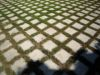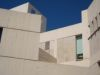# Lucas Borboleta

## Tag - espace

dimanche 1 décembre 2013

## Inner Product Space Characterization by Isometry Group over Unity Sphere

Why “power 2” in the Pythagorean theorem ?

Let us assume a geometry over the set E considered as 1) a vector space over the real field ; 2) with finite dimensions ; 3) equiped with a norm.

Which additional axioms have to be added in order to conclude that E is euclidean, or equivalently, that its norm is deriving from an inner product. Such additionnal axioms are searched in topology and in group formulations, as opposed to calculational ones, like the “polarisation identity”.

The attached document gathers theorems that proof the following axiom solution: 4) if a group G of isometries allows transportation between any pair of points of the unity sphere of E, then E is euclidean.

Borboleta_2013_Inner-Product-Space-Characterization-by-Isometric-Group-over-Unity-Sphere.pdfdimanche 24 novembre 2013

## Return on the 1932 proof of the Auerbach Theorem about Bounded Linear Groups

In 1932, Auerbach proved that, in any finite vector normed space, each bounded linear group left invariant a quadratic and positive form. This work revisits his proof with modern notation, and aims at paying attention to weaknesses: Borboleta_2013_Return-on-Auerbach-Theorem-for-bounded-linear-group.pdf.

mardi 16 octobre 2012

## Impressions de Matière et d'Espace à Barcelone

Voici, ramenées de Barcelone (Espagne), quelques impressions de Matière et d'Espace : Diaporama "Matière et Espace à Barcelone". Extrait :